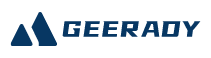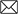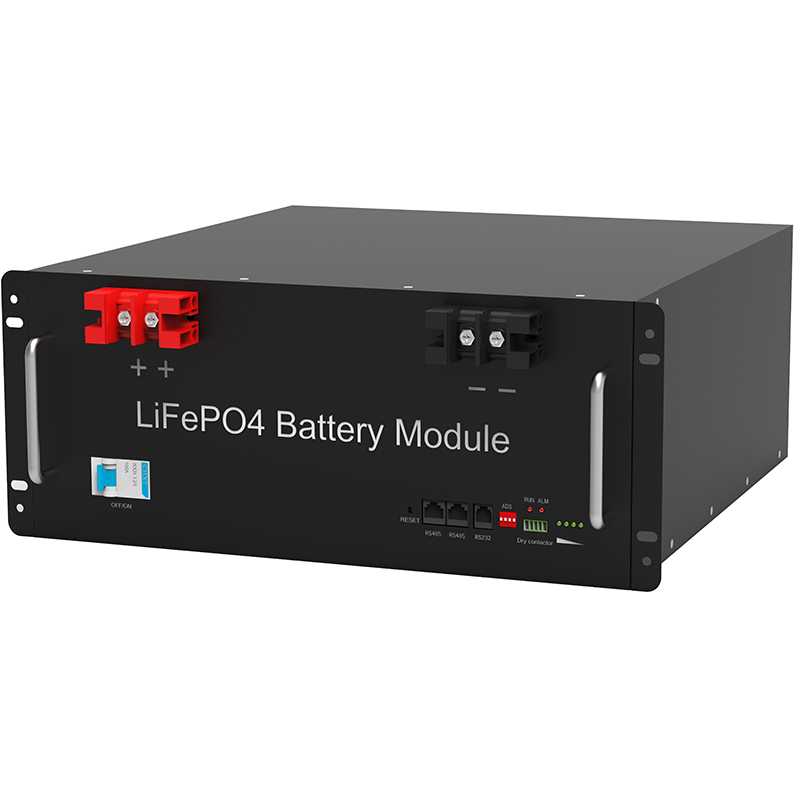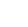#Emial:sales@geerady.com
Location： > > Battery work characteristics

### Battery work characteristics

Return Listsource：Gratene date： 2022-06-171. The valve controlled lead-acid battery consists of a positive, negative electrode plate, a partition, an electrolyte, a safety valve, and an outer casing, and the like.

In such a cathode absorption valve controlled lead-acid battery, the total amount of the negative electrode active material is 15%, and when the battery is charged, the positive electrode has been sufficient, the negative is not 90% of the capacity, so, under normal conditions, The positive electrode will produce oxygen, and the negative electrode does not produce hydrogen that is difficult to composite.

12V4AH battery

2. Battery indicator electromotive force, internal resistance, final voltage, discharge ratio, charging rate, cycle life.

(1) Battery electromotive force (E).

(2) Internal resistance (R) of the battery.

(3) Internal Voltage: The critical voltage when the battery-end voltage has dropped sharply, and if the discharge will damage the battery, the battery terminal voltage is called the final voltage. Different discharge rates have different discharges, U final = 1.66 + 0.0175h, in the formula H is discharge hour rate, such as 1 hour discharge ratio, U final = L.66 + 0.0175×1 = 1.68V, if used in 10 hours, U final = 1.66 + 0.0175×10 = 1.835V.

(4) Discharge Rate: The battery is slowly discharged to the final voltage under certain conditions. The size of the discharge current is represented by time rate and current. Usually used as discharge current at 10 hours. That is, the capacity of the battery is placed in the final voltage within 10 h. The battery capacity has changed as the discharge rate is changed, and when the discharge rate is lower than normal discharge, a large capacity can be obtained, and vice versa is reduced.

(5) Charging rate: The battery is called charging at a certain condition. The commonly used charging rate is 10 hours, that is, the charging period is reached after 10 hours of charging. When shortening the charging time, the charging current must increase, in turn, the charging current can be reduced.

(6) Circulating life: The battery has undergone charging and discharge, called a cycle. The number of cycles that the battery can withstand is referred to as a cycle life.

Recommend:

####TEL:

86-0755-36949293